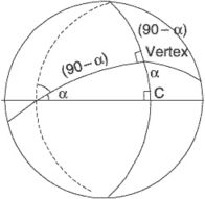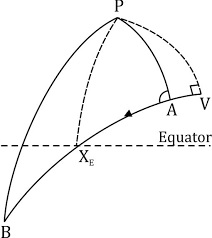# What is meaning of vertex of great circle sailing ?

The vertex of a great circle is the maximum latitude point of the great circle.###### The vertex has the following properties:

There is a maximum latitude point in both the northern and southern hemispheres; these points have the same value of latitude (eg if northern vertex = 40°N then southern vertex = 40°S). The longitudes of the vertices are 180° apart (e.g. if one is in 20°W, the other is in 160°E).

At the vertex the course on the great circle is exactly 090°T or 270°T, depending on whether you are proceeding towards the east or the west. This means that the angle between the great circle and the meridian at the vertex is always 90°.###### In other words we can define vertex as:

The vertex is the point on a great circle that is closest to the pole; by knowing the latitude of the vertex, if it is too high.

There are two vertices on a great circle, 180° apart; the nearer vertex is usually the chosen one for navigational calculation.

The vertex’s latitude is always numerically equal to or greater than the latitude of any other point on the great circle, including the latitude of departure and destination.

###### Note:

To find the position of the vertex you will first have to find the great circle initial course angle A. This will be found by the cosine rule. We will then know two parts of the triangle and can find any other part. The parts we know are Angle A and the Co-Latitude of A (PA).

We need to find PV (when taken from 90°, PV will give the latitude of the vertex), and angle VPA (the D.long between A and V) which is applied to the known longitude of A to give the longitude of the vertex.

Latitute of vertex:

Sin Mid Part= Cos opposite parts

Sin PV = (Cos Co A x Cos Co PA)

Sin PV = (Sin A x Sin PA)